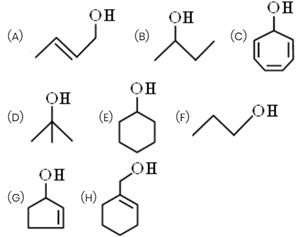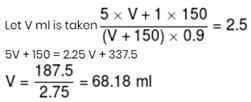Courses

# JEE Main Chemistry Mock

## 25 Questions MCQ Test Mock Test Series for JEE Main & Advanced 2021 | JEE Main Chemistry Mock

Description
This mock test of JEE Main Chemistry Mock for JEE helps you for every JEE entrance exam. This contains 25 Multiple Choice Questions for JEE JEE Main Chemistry Mock (mcq) to study with solutions a complete question bank. The solved questions answers in this JEE Main Chemistry Mock quiz give you a good mix of easy questions and tough questions. JEE students definitely take this JEE Main Chemistry Mock exercise for a better result in the exam. You can find other JEE Main Chemistry Mock extra questions, long questions & short questions for JEE on EduRev as well by searching above.
QUESTION: 1

Solution:
QUESTION: 2

Solution:
QUESTION: 3

### Identify the correct statement regarding enzymes.

Solution:
QUESTION: 4
The facor which changes equilibrium constant of the reaction A₂(g) + B₂(g) → 2AB(g) is
Solution:
QUESTION: 5
The ligand : NO is named as
Solution:
QUESTION: 6

Which one of the following reactions occur at the cathode?

Solution:
QUESTION: 7
The order of a reaction depends upon
Solution:
QUESTION: 8
The first ionisation potential of Li will be
Solution: As we move from left to right in a period, the ionisation potential increases with increasing atomic number and the ionisation potential keeps on decreasing regularly as we move down a group. Thus the first ionisation potential of Li is less than Be and higher than Na.
QUESTION: 9
Which of the following reactions actually take place?
Solution:
QUESTION: 10

When benzenediazonium chloride in hydrochloric acid reacts with cuprous chloride, then chlorobenzene is formed. The reaction is called

Solution:
QUESTION: 11
Which of the following is a non-electrolyte?
Solution:
QUESTION: 12
In decinormal solution, CH₃COOH acid is ionised to the extent of 1.3%. If log 1.3=0.11. What is the pH of the solution?
Solution:
QUESTION: 13

The Δ H value for the reaction H2 + Cl2  → 2 HCl is − 44.12 K.cal. If E1 is the activation energy of the reactants and E2 is the activation energy of the products, then for the above reaction

Solution:
QUESTION: 14
Phenolphthalein does not act as an indicator for the titration between
Solution:
QUESTION: 15
If H₂S is passed in ZnSO₄ containing HCl,Zinc sulphide is not precipitated because Ksp of
Solution:
QUESTION: 16
The reagent used to convert Ethanoic acid to Ethanol is
Solution:
QUESTION: 17

Conc. HNO3  forms NO2+  in presence of :

Solution:
QUESTION: 18
On mixing a certain alkane with chlorine and irradiating it with ultraviolet light,it forms only one monochloroalkane .This alkane could be
Solution:
QUESTION: 19
The solid NaCl is a bad conductor of electricity since
Solution:
QUESTION: 20

How many unit cells are present in a cube-shaped ideal crystal of NaCl of mass 1.0 g?(Atomic weights of Na = 23 and Cl = 35.5)

Solution:

Gram formula mass of NaCl = (23 + 35.5)g = 58.5g
Number of formula units of NaCl in 1.0g of NaCl = 6.02 x 1023/58.5 x1.0
There are four formula units per unit cell in NaCl
∴ Number of unit cells = 6.02 x 10 23 x1.0/58.5 x4 = 2.57 x 1021

*Answer can only contain numeric values
QUESTION: 21

How many alcohols give immediate turbidity on heating with Lucas Reagent?Solution:
*Answer can only contain numeric values
QUESTION: 22

If total number of chiral centre in α-D-Glucopyranose is 'P' and sucrose is 'Q' then what would be value of (P × Q)?

Solution:
*Answer can only contain numeric values
QUESTION: 23

At 25° C, the solubility product of Mg(OH)2 is 1.0 × 10–11. At which pH, will Mg2+ ions start precipitating in the form of Mg(OH)2 from a solution of 0.001 M Mg2+ ions ?

Solution:
*Answer can only contain numeric values
QUESTION: 24

Successive ionisation potential of an element are 7.6 ev, 12.9 ev, 27 ev, and 92.5 ev, then find number of electrons in the valence shell of the element.

Solution:
*Answer can only contain numeric values
QUESTION: 25

What volume in ml of 5 M H2SO4 should be added to 150 ml of 1 M H2SO4 to obtain a solution of molarity = 2.5 M, if upon mixing volume of solution decreases by 10% ?

Solution: